# introduction¶

Lettuce is an extremely useful and charming tool for BDD (Behavior Driven Development). It can execute plain-text functional descriptions as automated tests for Python projects, just as Cucumber does for Ruby.

Lettuce makes the development and testing process really easy, scalable, readable and - what is best - it allows someone who doesn’t program to describe the behavior of a certain system, without imagining those descriptions will automatically test the system during its development.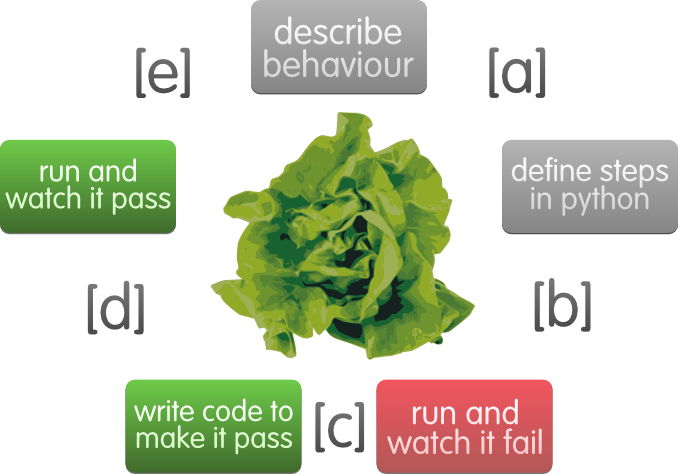# get lettuce¶

Make sure you’ve got Python installed and then run from the terminal:

```user@machine:~\$ [sudo] pip install lettuce
```

# define a problem¶

Let’s choose a problem to lettuce: Given a number, what is its factorial?

Note

The factorial of a positive integer n, denoted by n!, is the product of all positive integers less than or equal to n. The factorial of 0 is

# project structure¶

Build the directory tree bellow such as the files zero.feature and steps.py are empty.

```/home/user/projects/mymath
| tests
| features
- zero.feature
- steps.py
```

# lettuce it!¶

Lets begin to describe and solve our problem...

## first round¶

### [a] describe behaviour¶

Start describing the expected behaviour of factorial in zero.feature using English:

Feature: Compute factorial

In order to play with Lettuce As beginners We’ll implement factorial

Scenario: Factorial of 0
Given I have the number 0 When I compute its factorial Then I see the number 1

Note

zero.feature must be inside features directory and its extension must be .feature. However, you’re free to choose its name.

### [b] define steps in python¶

Now, let’s define the steps of the scenario, so Lettuce acan understand the behaviour description. Write steps.py file using Python:

```from lettuce import *

@step('I have the number (\d+)')
def have_the_number(step, number):
world.number = int(number)

@step('I compute its factorial')
def compute_its_fatorial(step):
world.number = factorial(world.number)

@step('I see the number (\d+)')
def check_number(step, expected):
expected = int(expected)
assert world.number == expected, \
"Got %d" % world.number

def factorial(number):
return -1
```

Note

steps.py must be inside features directory, but the names doesn’t need to be steps.py, it can be any .py terminated file, Lettuce will look for python files recursively within features dir.

Ideally, factorial will be defined somewhere else. However, as this is just a first example, we’ll implement it inside steps.py, so you get the idea of how to use Lettuce.

Notice that, until now, we haven’t defined the factorial function (it’s returning -1).

### [c] run and watch it fail¶

Go to the tests directory and run from the terminal:

```user@machine:~/projects/mymath/tests\$ lettuce
```

As you haven’t implemented factorial, it is no surprise the behavior won’t be reached: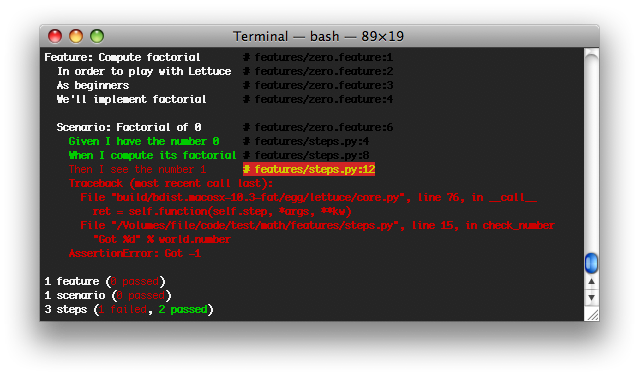Our only scenario failed :( Let’s solve it...

### [d] write code to make it pass¶

Well, by definition, we know that the factorial of 0 is 1. As our only feature is this... we could force factorial to return 1.

```from lettuce import *

@step('I have the number (\d+)')
def have_the_number(step, number):
world.number = int(number)

@step('I compute its factorial')
def compute_its_fatorial(step):
world.number = factorial(world.number)

@step('I see the number (\d+)')
def check_number(step, expected):
expected = int(expected)
assert world.number == expected, \
"Got %d" % world.number

def factorial(number):
return 1
```

### [e] run again and watch it pass¶

Again, run from the terminal:

```user@machine:~/projects/mymath/tests\$ lettuce
```

And you’ll be happy to see your factorial implementation passed all the behaviours expected: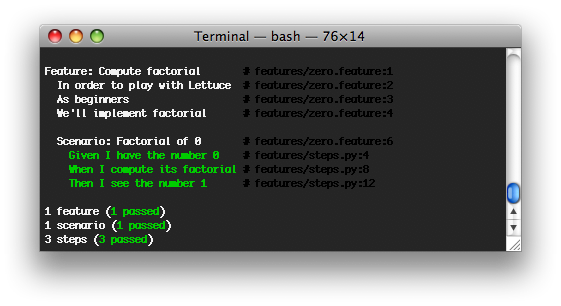Great! :)

However, one test is not enough for checking the quality of our solution... So let’s lettuce it again!

## second round¶

Let’s provide more tests so our problem is better described, and so we provide a more accurate implementation of factorial:

### [a] describe behaviour¶

Let’s provide two new scenarios, for numbers 1 and 2:

```Feature: Compute factorial
In order to play with Lettuce
As beginners
We'll implement factorial

Scenario: Factorial of 0
Given I have the number 0
When I compute its factorial
Then I see the number 1

Scenario: Factorial of 1
Given I have the number 1
When I compute its factorial
Then I see the number 1

Scenario: Factorial of 2
Given I have the number 2
When I compute its factorial
Then I see the number 2```

### [b] define steps in python¶

As we haven’t changed the definition, no need to make changes on this step.

### [c] run and watch it fail¶

```user@machine:~/projects/mymath/tests\$ lettuce
```

When running Letucce we realize that our previous implementation of factorial works fine both for 0 and for 1, but not for 2 - it fails. :(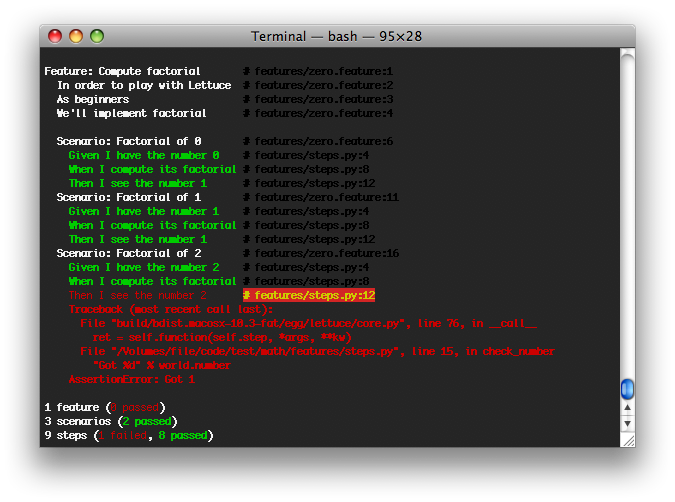### [d] write code to make it pass¶

Let’s provide a solution so we get the right factorial for all scenarions, specially for number 2:

```from lettuce import *

@step('I have the number (\d+)')
def have_the_number(step, number):
world.number = int(number)

@step('I compute its factorial')
def compute_its_fatorial(step):
world.number = factorial(world.number)

@step('I see the number (\d+)')
def check_number(step, expected):
expected = int(expected)
assert world.number == expected, \
"Got %d" % world.number

def factorial(number):
number = int(number)
if (number == 0) or (number == 1):
return 1
else:
return number
```

### [e] run again and watch it pass¶

```user@machine:~/projects/mymath/tests\$ lettuce
```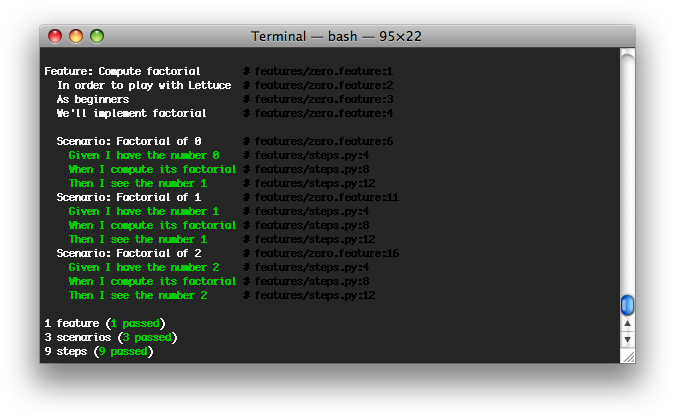Great! Three scenarios described and they are alright!

## third round¶

Let’s provide more tests so our problem is better described and we get new errors so we’ll be able to solve them.

### [a] describe behaviour¶

```Feature: Compute factorial
In order to play with Lettuce
As beginners
We'll implement factorial

Scenario: Factorial of 0
Given I have the number 0
When I compute its factorial
Then I see the number 1

Scenario: Factorial of 1
Given I have the number 1
When I compute its factorial
Then I see the number 1

Scenario: Factorial of 2
Given I have the number 2
When I compute its factorial
Then I see the number 2

Scenario: Factorial of 3
Given I have the number 3
When I compute its factorial
Then I see the number 6

Scenario: Factorial of 4
Given I have the number 4
When I compute its factorial
Then I see the number 24```

### [b] define steps in python¶

As we haven’t changed the definition, no need to make changes on this step.

### [c] run and watch it fail¶

```user@machine:~/projects/mymath/tests\$ lettuce
```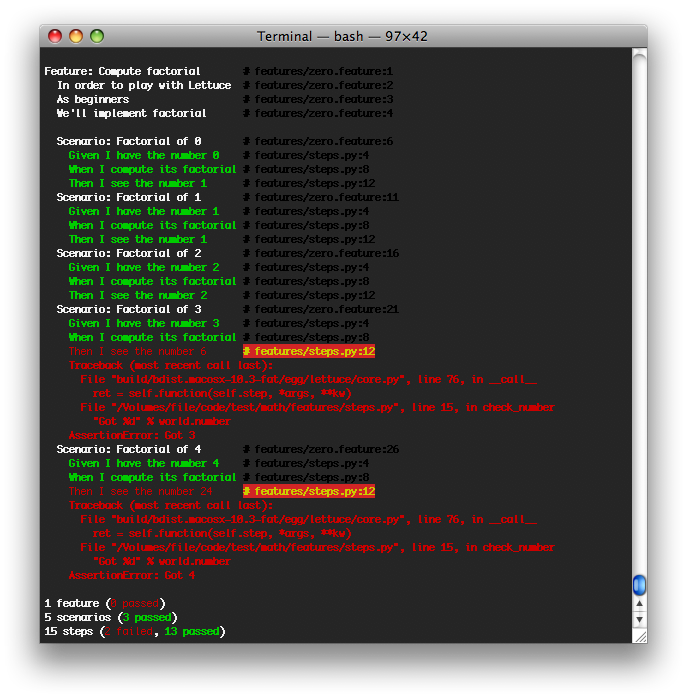### [d] write code to make it pass¶

```from lettuce import *

@step('I have the number (\d+)')
def have_the_number(step, number):
world.number = int(number)

@step('I compute its factorial')
def compute_its_fatorial(step):
world.number = factorial(world.number)

@step('I see the number (\d+)')
def check_number(step, expected):
expected = int(expected)
assert world.number == expected, \
"Got %d" % world.number

def factorial(number):
number = int(number)
if (number == 0) or (number == 1):
return 1
else:
return number*factorial(number-1)
```

### [e] run again and watch it pass¶

```user@machine:~/projects/mymath/tests\$ lettuce
```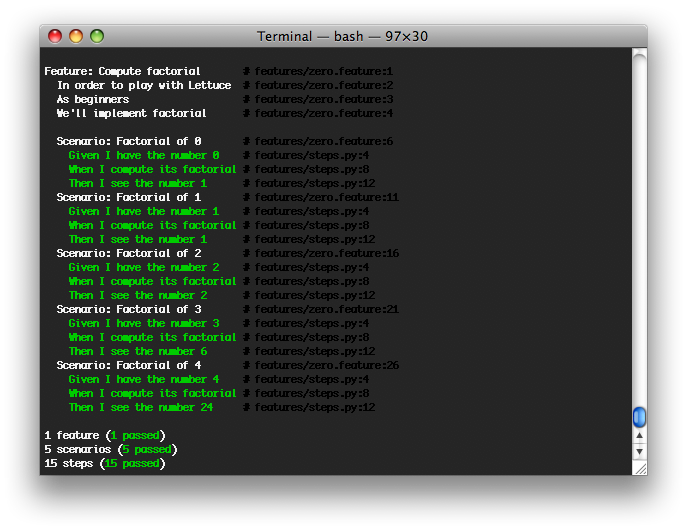## forth round¶

All steps should be repeated as long as you can keep doing them - the quality of your software depends on these.

Have a nice lettuce...! ;)